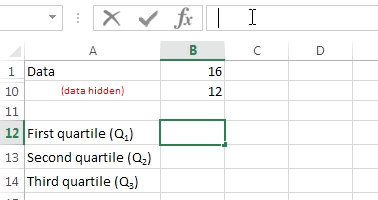### Excel formula

#### Summary Statistics (quartiles) | # gomaths.net/4235         User submitted, thanks to Simon Job

Calculate summary statistics: quartiles

First Quartile: `=QUARTILE(B1:B10,1)`

Second Quartile: `=QUARTILE(B1:B10,2)`

Third Quartile: `=QUARTILE(B1:B10,3)`

where `B1:B10` is the range of cells containing numerical data.

Note: Excel calculates quartiles differently to the usual method of splitting the data into 25% lots.